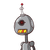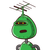# 3. The length of a football ground is 100 m and width 40 m. Findthe perimeter of the ground. Also find the cost of fencing i

3. The length of a football ground is 100 m and width 40 m. Find
the perimeter of the ground. Also find the cost of fencing it
with wire at the rate of 2.50 per metre.

### 2 thoughts on “3. The length of a football ground is 100 m and width 40 m. Find<br />the perimeter of the ground. Also find the cost of fencing i”

1.4000m=perimeter

fencing=2.5*4000=10000

Step-by-step explanation:

2.### Given:

• Length of the ground = 100 m
• Breadth of the ground = 40 m
• Cost of fencing it with wire is at the rate of ₹2.50 per metre.

### Tofind:

• Perimeter of the ground.
• Cost of fencing the ground.

[tex] \: [/tex]

### Solution:

As the dimensions of the football ground are different (100 m and 40 m), hence the ground is in rectangular shape. Therefore we’ll apply the formula for rectangle only.

We have to fence the ground, therefore we’ll find the perimeter.

[tex] \: [/tex]

[tex]\boxed {\mathfrak {\red {Perimeter\ of\ rectangle = 2(length\ +\ breadth)}}}[/tex]

Perimeter of ground = 2 (100+40)

Perimeter of ground = 2×140

Perimeter of ground = 280 m

[tex] \: [/tex]

Cost of fencing 1 m of ground = ₹2.50

Cost of fencing the entire ground = ₹ (280×2.50)

= ₹ 700

[tex] \: [/tex]